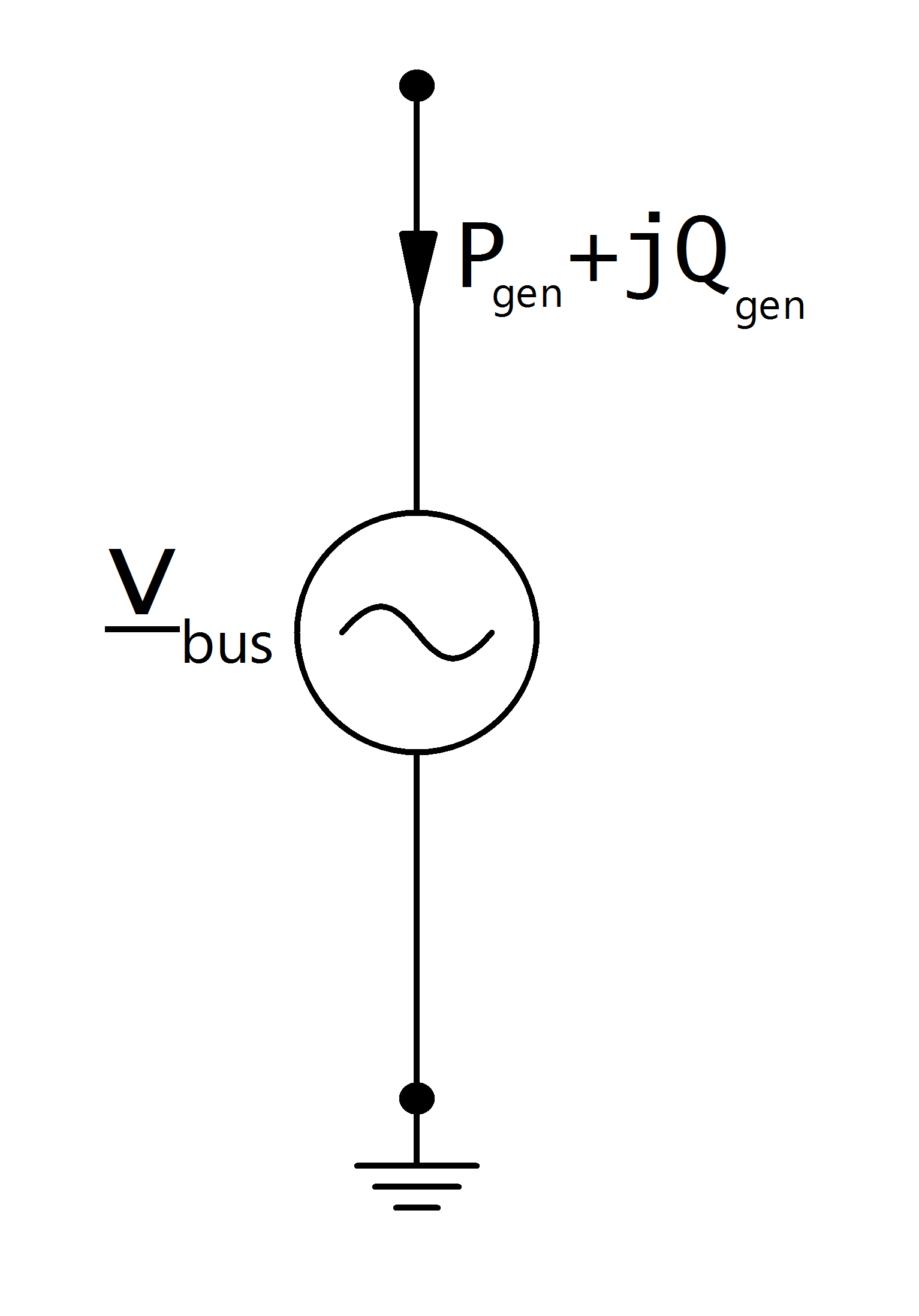Generator¶

Note

A generator with positive active power represents a voltage controlled generator. If you want to model constant generation without voltage control, use the Static Generator element.

Create Function¶

pandapower.create_gen(net, bus, p_mw, vm_pu=1.0, sn_mva=nan, name=None, index=None, max_q_mvar=nan, min_q_mvar=nan, min_p_mw=nan, max_p_mw=nan, scaling=1.0, type=None, slack=False, controllable=nan, vn_kv=nan, xdss_pu=nan, rdss_pu=nan, cos_phi=nan, in_service=True)

Adds a generator to the network.

Generators are always modelled as voltage controlled PV nodes, which is why the input parameter is active power and a voltage set point. If you want to model a generator as PQ load with fixed reactive power and variable voltage, please use a static generator instead.

INPUT:

net - The net within this generator should be created

bus (int) - The bus id to which the generator is connected

OPTIONAL:

p_mw (float, default 0) - The real power of the generator (negative for generation!)

vm_pu (float, default 0) - The voltage set point of the generator.

sn_mva (float, None) - Nominal power of the generator

name (string, None) - The name for this generator

index (int, None) - Force a specified ID if it is available. If None, the index one higher than the highest already existing index is selected.

scaling (float, 1.0) - scaling factor which for the active power of the generator

type (string, None) - type variable to classify generators

controllable (bool, NaN) - Whether this generator is controllable by the optimal powerflow

vn_kv (float, NaN) - Rated voltage of the generator for short-circuit calculation

xdss_pu (float, NaN) - Subtransient generator reactance for short-circuit calculation

rdss_pu (float, NaN) - Subtransient generator resistance for short-circuit calculation

cos_phi (float, NaN) - Rated cosine phi of the generator for short-circuit calculation

in_service (bool, True) - True for in_service or False for out of service

max_p_mw (float, default NaN) - Maximum active power injection - necessary for OPF

min_p_mw (float, default NaN) - Minimum active power injection - necessary for OPF

max_q_mvar (float, default NaN) - Maximum reactive power injection - necessary for OPF

min_q_mvar (float, default NaN) - Minimum reactive power injection - necessary for OPF

OUTPUT:
index (int) - The unique ID of the created generator
EXAMPLE:
create_gen(net, 1, p_mw = 120, vm_pu = 1.02)

Input Parameters¶

net.gen

 Parameter Datatype Value Range Explanation name string name of the generator type string naming conventions: “sync” - synchronous generator “async” - asynchronous generator type variable to classify generators bus* integer index of connected bus p_mw* float $$\leq$$ 0 the real power of the generator [MW] vm_pu* float voltage set point of the generator [p.u] sn_mva float $$>$$ 0 nominal power of the generator [MVA] min_q_mvar float minimal reactive power of the generator [MVar] max_q_mvar float maximal reactive power of the generator [MVar] scaling* float $$\leq$$ 0 scaling factor for the active power max_p_mw** float Maximum active power min_p_mw** float Minimum active power max_q_mvar** float Maximum reactive power min_q_mvar** float Minimum reactive power vn_kv*** float Rated voltage of the generator xdss_pu*** float $$>$$ 0 Subtransient generator reactance in per unit rdss_pu*** float $$>$$ 0 Subtransient generator resistence in per unit cos_phi*** float $$0 \leq$$ 1 Rated generator cosine phi in_service* boolean True / False specifies if the generator is in service.

*necessary for executing a power flow calculation
**optimal power flow parameter
***short-circuit calculation parameter

Electric Model¶

Generators are modelled as PV-nodes in the power flow:Voltage magnitude and active power are defined by the input parameters in the generator table:

\begin{align*} P_{gen} &= p\_mw * scaling \\ v_{bus} &= vm\_pu \end{align*}

Result Parameters¶

net.res_gen

 Parameter Datatype Explanation p_mw float resulting active power demand after scaling [MW] q_mvar float resulting reactive power demand after scaling [MVar] va_degree float generator voltage angle [degree] vm_pu float voltage at the generator [p.u]

The power flow returns reactive generator power and generator voltage angle:

\begin{align*} p\_mw &= P_{gen} \\ q\_mvar &= Q_{gen} \\ va\_degree &= \angle \underline{v}_{bus} \\ vm\_degree &= |\underline{v}_{bus}| \end{align*}

Note

If the power flow is run with the enforce_qlims option and the generator reactive power exceeds / underruns the maximum / minimum reactive power limit, the generator is converted to a static generator with the maximum / minimum reactive power as constant reactive power generation. The voltage at the generator bus is then no longer equal to the voltage set point defined in the parameter table.